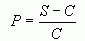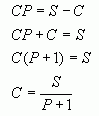From: Purushotham, a parent Question: If the selling price is 120 and the profit is 20%, what is the cost price? Hi. If you subtract the selling price from the cost price, you get the amount of profit (or loss) for the item. If you divide this by the cost price, you get the percentage of profit (or loss). If S = selling price and C = cost price and you have the percentage profit P as a decimal (remember that 20% = 0.20), then that meansYou have S (it is Rs. 120) and P (it is 0.20) and you want to find C. That means you need to re-arrange this equation to solve for C. Here are the steps to do that:Now you can sustitute your numbers in for S and P and you can find the cost price C. Hope this helps! Stephen La Rocque.